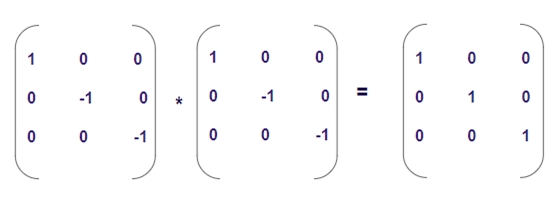# Program to check Involutory Matrix in C++

Given a matrix M[r][c], ‘r’ denotes number of rows and ‘c’ denotes number of columns such that r = c forming a square matrix. We have to check whether the given square matrix is an Involutory matrix or not.

## Involutory Matrix

A matrix is called Involutory matrix if and only if, when a matrix gets multiplied with itself and its result is an identity matrix. A matrix I is Identity matrix if and only if its main diagonal is one and other elements than the main diagonal are zero. So, we can say a matrix is Involutory matrix if and only if M*M=I, where M is some matrix and I is an Identity Matrix.

Like in the given Example below −Here when we multiplied the matrix with itself the result is the identity matrix; hence the given matrix is Involutory Matrix.

## Example

Input: { {1, 0, 0},
{0, -1, 0},
{0, 0, -1}}
Output: yes
Input: { {3, 0, 0},
{0, 2, 0},
{0, 0, 3} }
Output: no

## Algorithm

Start
Step 1 -> define macro as #define size 3
Step 2 -> declare function for matrix multiplication.
void multiply(int arr[][size], int res[][size])
Loop For int i = 0 and i < size and i++
Loop For int j = 0 and j < size and j++
Set res[i][j] = 0
Loop For int k = 0 and k < size and k++
Set res[i][j] += arr[i][k] * arr[k][j]
End
End
End
Step 3 -> declare function to check involutory matrix or not
bool check(int arr[size][size])
declare int res[size][size]
Call multiply(arr, res)
Loop For int i = 0 and i < size and i++
Loop For int j = 0 and j < size and j++
IF (i == j && res[i][j] != 1)
return false
End
If (i != j && res[i][j] != 0)
return false
End
End
End
Return true
Step 4 -> In main()
Declare int arr[size][size] = { { 1, 0, 0 },
{ 0, -1, 0 },
{ 0, 0, -1 } }
If (check(arr))
Print its an involutory matrix
Else
Print its not an involutory matrix
Stop

## Example

#include <bits/stdc++.h>
#define size 3
using namespace std;
// matrix multiplication.
void multiply(int arr[][size], int res[][size]){
for (int i = 0; i < size; i++){
for (int j = 0; j < size; j++){
res[i][j] = 0;
for (int k = 0; k < size; k++)
res[i][j] += arr[i][k] * arr[k][j];
}
}
}
// check involutory matrix or not.
bool check(int arr[size][size]){
int res[size][size];
multiply(arr, res);
for (int i = 0; i < size; i++){
for (int j = 0; j < size; j++){
if (i == j && res[i][j] != 1)
return false;
if (i != j && res[i][j] != 0)
return false;
}
}
return true;
}
int main(){
int arr[size][size] = { { 1, 0, 0 },
{ 0, -1, 0 },
{ 0, 0, -1 } };
if (check(arr))
cout << "its an involutory matrix";
else
cout << "its not an involutory matrix";
return 0;
}

## Output

its an involutory matrix